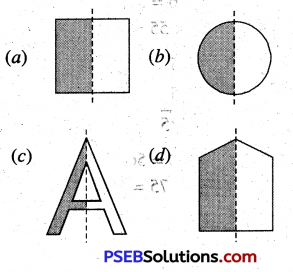# PSEB 4th Class Maths Solutions Chapter 10 Patterns Ex 10.1

Punjab State Board PSEB 4th Class Maths Book Solutions Chapter 10 Patterns Ex 10.1 Textbook Exercise Questions and Answers.

## PSEB Solutions for Class 4 Maths Chapter 10 Patterns Ex 10.1

Question 1.
Observe the pattern carefully and complete it.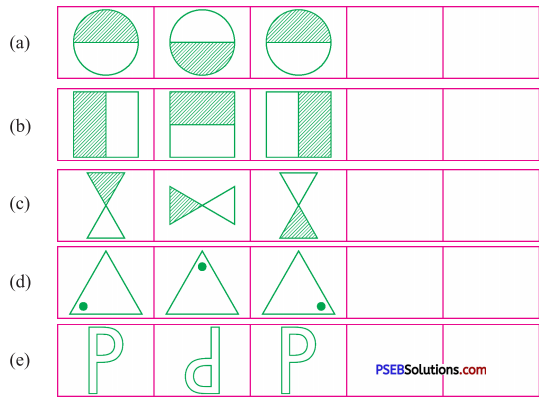Solution: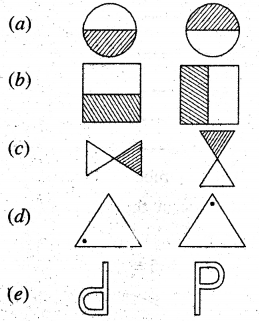Question 2.
Students of a particular school who have liking for different types of fruits.
(a) 9981
(b) 6039
(c) 243
(d) 6308
(e) 6415
(f) 9108
(g) 1728
(h) 8714
(i) 53694
(j) 40819.
Solution:
(a) 9981
First of all omit 9’s from the given number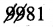Remaining digits of number = 8,1
Now add the remaining• digits e.
= 8, 1
8 + 1 = 9
omit this 9
Now there is no digit left’
Hence, this number is a multiple of 9
Second Method: 9981
Sum of the digits = 9 + 9 + 8 + 1 = 27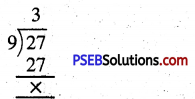Because, sum of digits is divisible by 9
Hence, this number is a multiple of 9

(b) 6039
Sum of the digits = 6 + 0 + 3 + 9 = 18
Because sum of digits is divisible by 9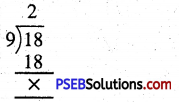Hence, this number is multiple of 9.(c) 243
Sum of the digits = 2 + 4 + 3 = 9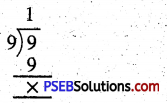Because, sum of digits is divisible by 9
Hence, this number is a multiple of 9

(d) 6308
Sum of the digits = 6 + 3 + 0 + 8 = 17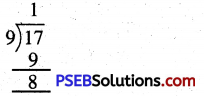Because sum of digits is not divisible by 9
Hence, this number is not a multiple of 9
(e) 6415
Sum of the digits = 6 + 4 + 1 + 5 = 16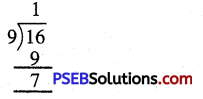Because, sum of digits is not divisible by 9
Hence, this number is not a multiple of 9

(f) 9108
Sum of the digits = 9 + 1 + 0 + 8 = 18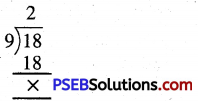Because sum of digits is divisible by 9
Hence, this number is a multiple of 9

(g) 1728
Sum of the digits = 1 + 7 + 2 + 8 = 18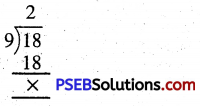Because sum of digits is divisible by 9
Hence, this number is a multiple of 9

(h) 8714
Sum of the digits = 8 + 7 + 1 + 4 = 20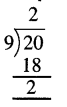Because sum of digits is divisible by 9
Hence, this number is a multiple of 9

(i) 53694
Sum of the digits = 5 + 3 + 6 + 9 + 4 = 27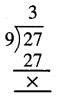Because sum of digits is divisible by 9
Hence, this number is a multiple of 9(j) 40819.
Sum of the digits = 4 + 0 + 8 + 1 + 9 = 22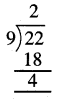Because sum of digits is not divisible by 9
Hence, this number is nòt a multiple of 9

Question 3.
Multiply the given numbers using pattern:
(a) 35 × 10 = ………
(b) 9 × 10 = ………
(c) 21 × 10 = ……..
(d) 106 × 10 = ……..
(e) 148 × 10 = ………
(f) 2 × 1oo = ……….
(g) 20 × 1oo = ………
(h) 38 × 100 = ……..
(i) 209 × 100 = ……..
(j) 406 × 100 = ………
Solution:
(a) 350,
(b) 90,
(c) 210,
(d) 1060,
(e) 1480,
(f) 200,
(g) 2000,
(h) 3800,
(j) 20900,
(j) 40600.

Question 4.
Divide the given numbers (Using patterns) :
(a) 60 ÷ 10 = ………..
(b) 700 ÷ 10 = ……..
(c) 96 ÷ 10 = ……….
(d) 600 ÷ 100 = ……….
(e) 1500 ÷ 100 = ……….
(f) 1000 ÷ 100 = …….
Solution:
(a) 6,
(b) 70,
(c) 96,
(d) 6,
(e) 15,
(f) 10.

Question 5.
Fill in the blanks:
(a) …….. × 10 = 500
(b) …… ÷ 10 = 96
(c) ……. × 100 = 900
(d) …….. ÷ 100 = 7
Solution:
(a) 50,
(b) 960,
(c) 9,
(d) 700.

Question 6.
Complete the following towers: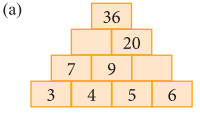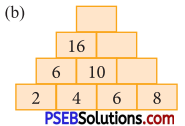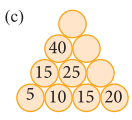Solution:
(a) 5 + 6 = 11, 7 + 9 = 16
(b) 6 + 8 = 14, 10 + 14 = 24, 16 + 24 = 40
(c) 15 + 20 = 35, 25 + 35 = 60, 40 + 60 = 100.Question 7.
Multiply the given numbers in one line: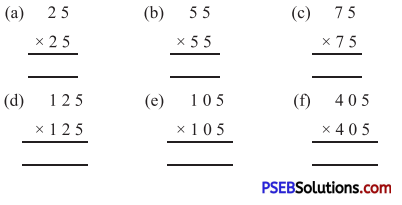Solution: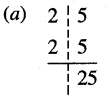2 × 3 = 6
∴ 25 × 25 = 625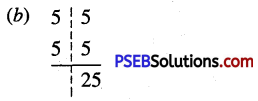5 × 6 = 30
∴ 55 × 55 = 3025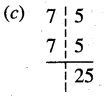7 × 8 = 56
∴ 75 × 75 = 5625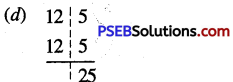12 × 13 = 156
∴ 125 × 125 = 15625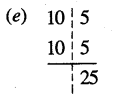10 × 11 = 110
∴ 105 × 105 = 11025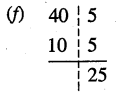40 × 41 = 1640
∴ 405 × 405 = 164025

Question 8.
Solve:
(a) (13 × 13) – (12 × 12)
(b) (18 × 18) – (17 × 17)
(c) (35 × 35) – (34 × 34)
(d) (120 × 120) – (119 × 119)
(e) (151 × 151) – (150 × 150)
solution:
(a) 13 + 12 = 25,
(b) 18 + 17 = 35,
(c) 35 + 34 = 69,
(d) 120 + 119 = 239,
(e) 151 + 150 = 301.

Question 9.
Complete the series according to pattern:
(a) 1 + 2 + 3 + 4 + 5 + 6 + 7 + 8 + 9 + 10 = 55
11 + 12 + 13 + 14 + …….. + 19 + 20 = 155
21 + 22 + 23 + 24 + …… + 29 + 30 = 255
31 + 32 + 33 + 34 + …… + 39 + 40 = ………..
41 + 42 + 43 + 44 + …….. + 49 + 50 = ………..
51 + 52 + 53 + 54 + …….. + 59 + 60 = ……….

(b) 1 × 1 = 1
11 × 11 = 121
111 × 111 = 12321
1111 × 1111 = 1234321
11111 × 11111 = ……….
111111 × 111111 = ……….
111111 × 111111 = ………..
Solution:
(a) 355, 455, 555,
(b) 123454321, 12345654321, 1234567654321.

Question 10.
Find an easy method to complete the series. Write next three numbers using this method:
(a) 7, 12, 17, ……, …….., ………..
(b) 2, 4, 8, ……., ………., ………..
(c) 100, 90, 80, ……., ………., ………..
(d) 66, 55, 44, ……., ………., ………..
(e) 108, 208, 308, ……., ………., ………..
(f) 40, 39, 38, ……., ………., ………..
Solution:
(a) 22, 27, 32,
(b) 16, 32, 64,
(c) 70, 60, 50,
(d) 33, 22, 11,
(e) 408, 508, 608
(f) 37, 36, 35

Question 11.
Draw symmetry lines in the following: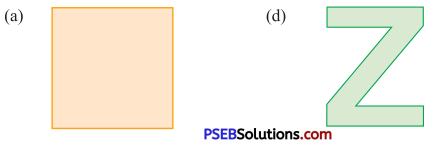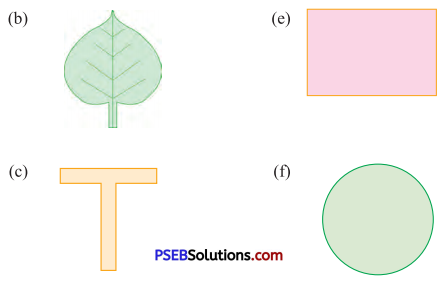Solution: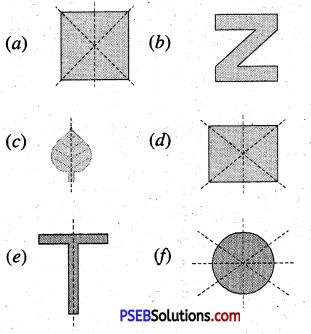Question 12.
Complete the given symmetrical patterns: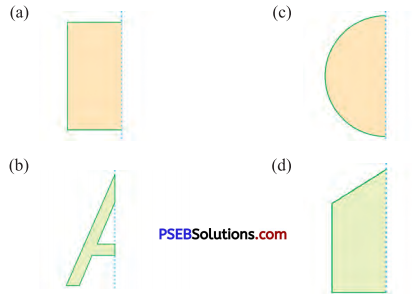Solution: